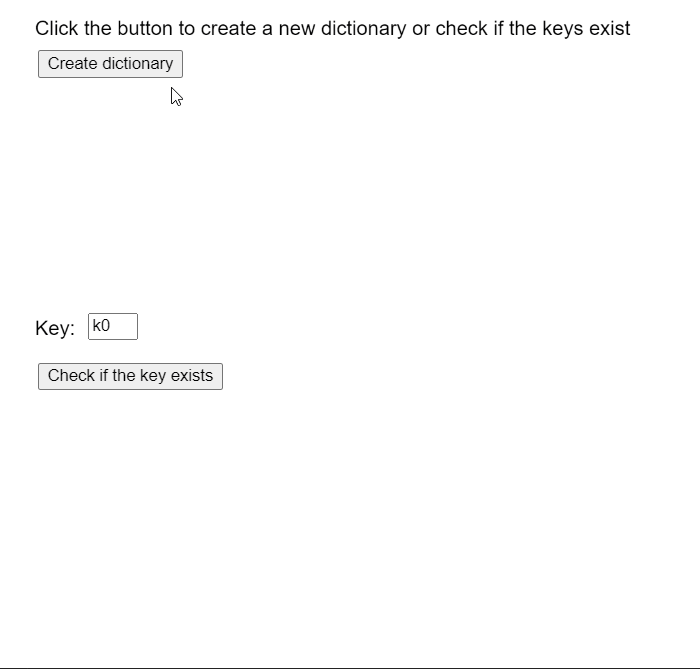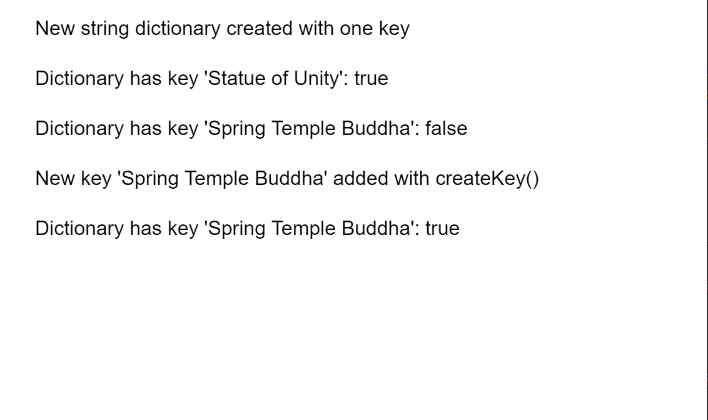• Last Updated : 25 Nov, 2020

The hasKey() method of p5.TypedDict in p5.js is used to check if the given key exists in the typed dictionary. This method returns true if the given key exists, else it returns false. A key-value pair is a set of two values that are mapped to each other. These values can be accessed by querying this dictionary using the key portion of the pair. A typed dictionary can store multiple key-value pairs that can be accessed using the methods of the dictionary.

Syntax:

Hey geek! The constant emerging technologies in the world of web development always keeps the excitement for this subject through the roof. But before you tackle the big projects, we suggest you start by learning the basics. Kickstart your web development journey by learning JS concepts with our JavaScript Course. Now at it's lowest price ever!

```hasKey( key )
```

Parameters: This method accepts a single parameter as shown above and discussed below:

• key: This is a number that denotes the key that has to be checked in the dictionary.

The examples below illustrate the hasKey() method in p5.js:

Example 1:

## Javascript

 `let y = 0;`` ` `function` `setup() {``  ``createCanvas(550, 500);``  ``textSize(16);`` ` `  ``text(``"Click the button to create a new "` `+``       ``"dictionary or check if the keys exist"``,``       ``20, 20);`` ` `  ``text(``"Key:"``, 20, 260);`` ` `  ``key_input = createInput(``'k0'``);``  ``key_input.position(70, 250);``  ``key_input.size(40);`` ` `  ``createBtn = createButton(``"Create dictionary"``);``  ``createBtn.position(30, 40);``  ``createBtn.mouseClicked(createNewDict);`` ` `  ``checkBtn = createButton(``"Check if the key exists"``);``  ``checkBtn.position(30, 290);``  ``checkBtn.mouseClicked(checkVal);``}`` ` `function` `createNewDict() {``  ``clear();`` ` `  ``// Create an object with random values``  ``let obj = {};``  ``for` `(let i = 0; i < 5; i++) {``    ``let rk = ``"k"` `+ i;``    ``let rn = ``"v"` `+ i;``    ``obj[rk] = rn;`` ` `    ``text(``"Key: "` `+ rk + ``"  Value: "` `+``         ``rn, 40, 120 + 20 * i);``  ``}`` ` `  ``// Create a string dict using the above values``  ``numDict = createStringDict(obj);`` ` `  ``text(``"New Dictionary created with values"``,``       ``20, 80);`` ` `  ``text(``"Click the button to create a new "` `+``       ``"dictionary or check if the keys exist"``,``       ``20, 20);`` ` `  ``text(``"Key:"``, 20, 260);``}`` ` `function` `checkVal() {`` ` `  ``// Get the key to be checked``  ``let keyToCheck = key_input.value();`` ` `  ``// Use hasKey() to check if the key exists``  ``let hasEntry = numDict.hasKey(keyToCheck);`` ` `  ``// If the key exists in the dictionary``  ``if` `(hasEntry) {`` ` `    ``let keyVal = numDict.get(keyToCheck);``   ` `    ``text(``"The value at key: "` `+ keyToCheck +``         ``" is: "` `+ keyVal, 20, 340 + y * 20);``  ``}`` ` `  ``// The key does not exist``  ``else` `{``    ``text(``"The key does not exist"``,``         ``20, 340 + y * 20);``  ``}``  ``y++;`` ` `  ``text(``"Click the button to create a new "` `+``       ``"dictionary or check if the keys exist"``,``       ``20, 20);`` ` `  ``text(``"Key:"``, 20, 260);``}`

Output:Example 2:

## Javascript

 `function` `setup() {``  ``createCanvas(550, 300);``  ``textSize(16);`` ` `  ``let stringDict =``      ``createStringDict(``'Statue of Unity'``,``                       ``'182 m'``);``  ``text(``"New string dictionary created "` `+``       ``"with one key"``, 20, 20);`` ` `  ``// Check if the specified key exists``  ``let existOne =``      ``stringDict.hasKey(``'Statue of Unity'``);``  ``text(``"Dictionary has key 'Statue of Unity': "` `+``       ``existOne, 20, 60);`` ` `  ``let existTwo =``      ``stringDict.hasKey(``'Spring Temple Buddha'``);``  ``text(``"Dictionary has key "` `+``       ``"'Spring Temple Buddha': "` `+``       ``existTwo, 20, 100);`` ` `  ``// Add the given key to the dictionary``  ``// specifying the key and value``  ``stringDict.create(``'Spring Temple Buddha'``,``                    ``'128 m'``);``  ``text(``"New key 'Spring Temple Buddha' "` `+``       ``"added with createKey()"``, 20, 140)`` ` `  ``// Check if the specified key exists again``  ``existTwo =``    ``stringDict.hasKey(``'Spring Temple Buddha'``);``  ``text(``"Dictionary has key "` `+``       ``"'Spring Temple Buddha': "` `+``       ``existTwo, 20, 180);``}`

Output:Online editor: https://editor.p5js.org/
Environment Setup: https://www.geeksforgeeks.org/p5-js-soundfile-object-installation-and-methods/